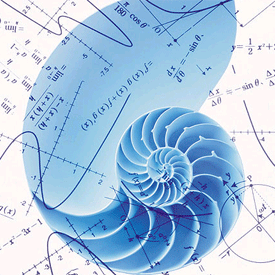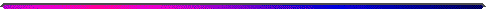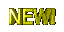Vancouver Island University

Department of Mathematics

Instructor Dr. Lev V. Idels

MATH -370: Elementary Numerical Mathematics

Spring 2020General Course Information

Elementary techniques of numerical solution of mathematical problems: solution of algebraic equations, finite differences, interpolation, systems of equations, numerical differentiation and integration, curve-fitting by splines, and numerical methods for initial-value problems. (3:0:0)

Prerequisite: Six credits of 200-level Mathematics courses (excluding Math 203). Additional prerequisites, depending on the topic to be studied, may be in effect.

Course objectives:

Numerical analysis is the story of how functions, derivatives, integrals, and differential equations are handled as strings of numbers in the computer. This course analyzed the basic techniques for the efficient numerical solution of problems in science, economics and engineering. Topics spanned root finding, interpolation and approximation of functions, integration, differential equations, and iterative methods in linear algebra. You will also learn to use MATLAB in order to explore and apply the mathematical techniques taught in the lectures. A few class lectures will be devoted to MATLAB which will suffice in case students have not had prior programming experience.

Topics (Tentative)

Prelude. Error Analysis. Algorithms and Convergence. Numerical Methods for Nonlinear equations.

Assignment & Homework #1.Due Mon Feb 3 2014

Numerical approximation of functions by simpler computational building blocks (for example - polynomials and splines). Curve fitting.

Assignment & Homework #2. Due March 2

Numerical differentiation and integration (Richardson, Romberg and Gaussian Methods).

Assignment  & Homework #3. Due March 19

Numerical solutions of ordinary differential equations and boundary value problems.

Assignment & Homework #4. Due March 31

Numerical Methods for Linear Algebra. Iterative techniques. Ill-conditional Systems.

Assignment & Homework #5. Due April 14

No textbook is required for this course. Lecture notes will not, in general, be provided.If you choose to take this class, you’ll need to attend. Don’t decide to take this class without committing yourself to attending each and every class.

40%      5 Assignments & Homework

20%      Midterm Test March 19

40%      Final examWarning:

In the case that the completed assignments of two students are found to be principally copied from each other, the corresponding assignments will not be counted for each of the two students involved, and the cases have to be reported to the university for penalty according to the university's regulations

Class etiquette: Use of cell phones (including text messaging, answered or unanswered ringing etc.), laptops, pagers, Blackberries and most other electronic gizmos during class is highly inappropriate and disrespectful to both the instructor and fellow students. Chit chat with neighbors, even when whispered, is equally unacceptable.

Office: Building: 359 Room: 204 Ph: 7533245 Local 2429

Office hours: TBA or if you need to see me outside of the announced office hours, please set up an appointment with me, either by speaking to me before or after class, or by sending me an email message (include "MATH 370 in the subject line, please).

To help me manage my email inbox, please include "MATH 370" in the subject line of any email message you send to me (without it, your message runs the risk of being deleted without being read).

ENJOY THE COURSE!